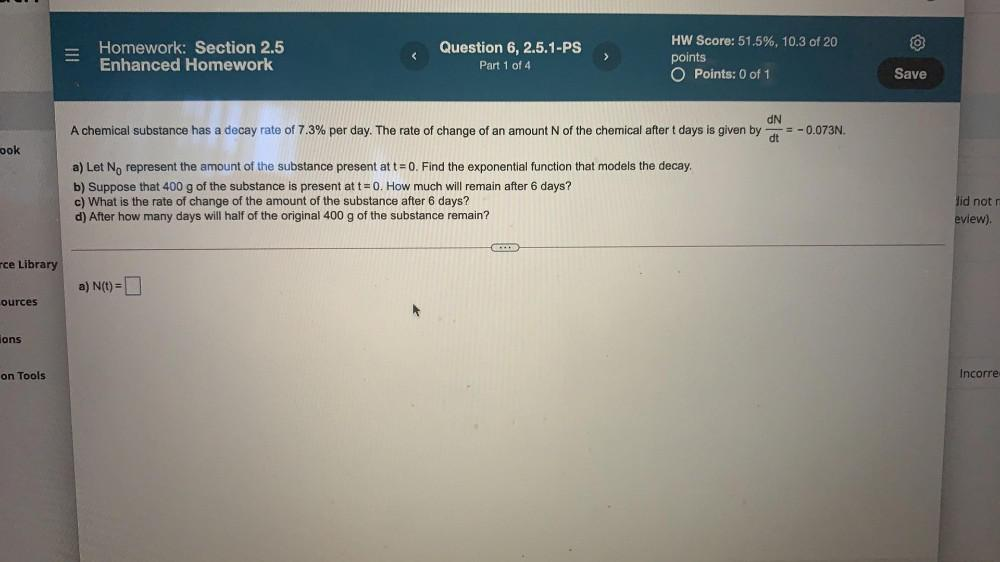Question:

# Homework: Section 2.5 Enhanced Homework Question 6, 2.5.1-PS Part 1 of 4 > HW Score: 51.5%, 10.3 of 20 points O Points: 0 of 1 SHomework: Section 2.5 Enhanced Homework Question 6, 2.5.1-PS Part 1 of 4 > HW Score: 51.5%, 10.3 of 20 points O Points: 0 of 1 Save A chemical substance has a decay rate of 7.3% per day. The rate of change of an amount N of the chemical after t days is given by dN = -0.073N. dt ook a) Let No represent the amount of the substance present at t = 0. Find the exponential function that models the decay. b) Suppose that 400 g of the substance is present at t= 0. How much will remain after 6 days? c) What is the rate of change of the amount of the substance after 6 days? d) After how many days will half of the original 400 g of the substance remain? did not eview) rce Library a) N(t)= cources A ions on Tools Incorre